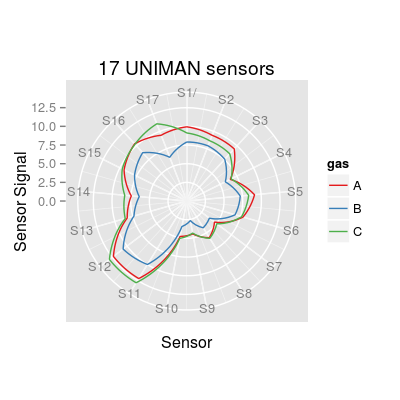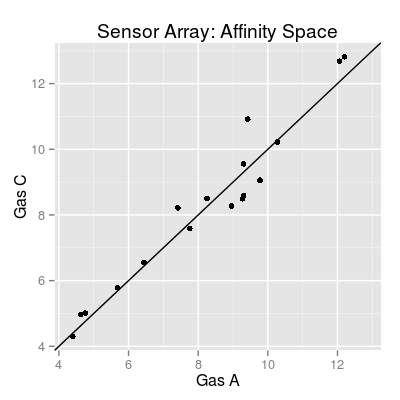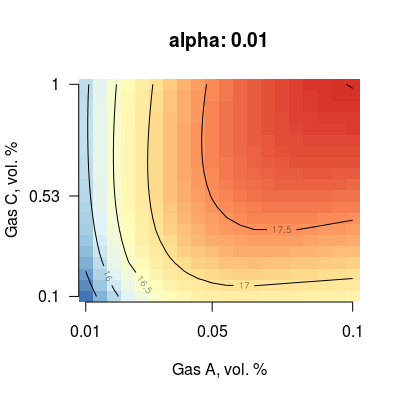Demo NeuromorphicSim.

Description

This demonstration shows an example of neuromorphic simulations possible with package chemosensors-package. Particularly, we perform a mixture quantification scenario, where the system is trained on pure analytes and validation is thought to quantify concentration of components in mixtures.

Details

The user should be aware of the following issues when implementing such simulations.

• A concentration matrix (of three analytes maximum) can be coded in several ways, manually by function matrix or by using methods of class Scenario. See the example code on class help page Scenario for more details.
• To configure a sensor array, there are a list of parameters for initialization method of class SensorArray: the number of sensors (nsensors), sensor types (num), sensor non-linearity (alpha), sensor diversity (beta), noise levels (csd, ssd and dsd), and sensor dynamics (enableDyn).
• To parallelize the computation in data generation process, one can specify the parameter nclusters (default value is 1) of method predict. Another alternative is to set a global option cores by command options(cores = 2).

Examples

# Many lines of code are commented to respect the CRAN policy on CPU time per Rd file. # options(cores = 2) # use this option to confiure the parallel computation # (in this case 2 CPU cores are specified) ### step 1/7: take a look at the reference array of 17 UNIMAN sensors sa.uniman <- SensorArray(nsensors = 17) # polar plot plot(sa.uniman, 'polar', main = "17 UNIMAN sensors")# affinity space for gases 1 and 3 plot(sa.uniman, 'affinitySpace', gases = c(1, 3))### step 2/7: select sensor non-linearity (parameter alpha) for a sensor # - (here) sensor `num` is 3, tesed values of `alpha` are 0.01, 1 and 2.25 # - (note) in the current implementation, parameter `alpha` is the same # for all sensors in a array s <- Sensor(num = 3, alpha = 0.01) plotMixture(s, gases = c(1, 3), main = paste("alpha:", alpha(s)))#s <- Sensor(num = 3, alpha = 1) #plotMixture(s, gases = c(1, 3), main = paste("alpha:", alpha(s))) #s <- Sensor(num = 3, alpha = 2.25) #plotMixture(s, gases = c(1, 3), main = paste("alpha:", alpha(s))) ### step 3/7: select sensor diversity (parameter beta) for a sensor array # - (here) the number of sensors `nsensors` is 34 (two times more than 17 UNIMAN sensors) # tesed values of `beta` are 1, 2, 5 and 10 sa <- SensorArray(nsensors = 34, beta = 1) plot(sa, 'polar', main = paste("beta:", beta(sa)))#sa <- SensorArray(nsensors = 34, beta = 2) #plot(sa, 'polar', main = paste("beta:", beta(sa))) #sa <- SensorArray(nsensors = 34, beta = 5) #plot(sa, 'polar', main = paste("beta:", beta(sa))) #sa <- SensorArray(nsensors = 34, beta = 10) #plot(sa, 'polar', main = paste("beta:", beta(sa))) ### step 4/7: define the parameters of the array # - (here we use the default parameters) # `alpha` is 2.25 (default), `beta` is 2, # noise parameters `csd`, `ssd` and `dsd` are all 0.1, sa <- SensorArray(nsensors = 34) # polar plot #plot(sa, 'polar', main = "Virtual sensors") # affinity space for gases 1 and 3 #plot(sa, 'affinitySpace', gases = c(1, 3)) ### step 5: define a concentration matrix (via class Scenario) # - (here) let's assume a scenario of mixture quantification for gases 1 and 3 # `tunit` is set to 60 (recommended value) training.set <- c("A 0.01", "A 0.05", "C 0.1", "C 1") validation.set <- c("A 0.01, C 0.1", "A 0.0025, C 0.5", "A 0.05, C 1") sc <- Scenario(tunit = 60, T = training.set, nT = 2, V = validation.set, nV = 2) #plot(sc) #plot(sc, facet = FALSE, concUnits = 'norm') # extract conc. matrix conc <- getConc(sc) print(head(conc))
A B C 1 0 0 0 2 0 0 0 3 0 0 0 4 0 0 0 5 0 0 0 6 0 0 0
### step 6/7: generate sensor data in reaction to `conc` conc0 <- conc[1:240, ] # to save CPU time of demonstration, # we use just two cycles of 2x60 time length each # 1 cycle = gas exposure phase and cleaning phase #sdata <- predict(sa, conc0, nclusters = 2) #p1 <- qplot(X1, value, data = melt(sdata), geom = "line") + facet_wrap(~ X2) + # xlab("Time, a.u.") + ylab("Sensor Signal, a.u.") ### step 7/7: some additional plots for testing # plot just few sensors #p2 <- qplot(X1, value, data = melt(sdata[, 1:2]), geom = "line") + facet_wrap(~ X2) + # labs(x = "Time, a.u.", y = "Sensor Signal, a.u.", title = "First two sensors") # re-generate sensor data as noise-free sa0 <- sa csd(sa0) <- 0 ssd(sa0) <- 0 dsd(sa0) <- 0 sa0
Sensor Array of 34 sensors, 3 gases A, B, C - enableSorption TRUE, enableDyn FALSE - Sensor Model (num 1, 2, 3 ... 17), beta 2, data model 'ispline' - Sorption Model (knum 1, 2, 3 ... 17), alpha 2.25 - Concentration Noise Model (csd 0), noise type 'logconc' - Sensor Noise Model (ssd 0), noise type 'randomWalk' - Drift Noise Model (dsd 0), common model 'cpc'
#sdata0 <- predict(sa0, conc0, nclusters = 2) #p3 <- qplot(X1, value, data = melt(sdata0), geom = "line") + facet_wrap(~ X2) + # labs(x = "Time, a.u.", y = "Sensor Signal, a.u.", title = "Noise-free array")